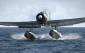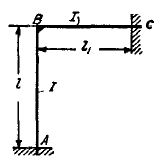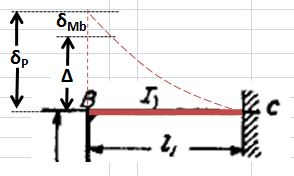Problem: A frame consists of two bars joined rigidly at B and built in
at A and C (see Figure). Determine the bending moment M at B
and the compressive force P in AB when, due to a rise in temperature,
the bar AB increases in length byAnswer. P and M can be found from the equations:**************************************************************************
**************************************************************************
My Solution: (I was able to solve the 1st equation in the answer above, however, I am having too much difficulty getting the 2nd equation, kindly see my solution below... anu kaya ang maling ginagawa ko? sensya na po...)

For the 1st equation, consider beam BC as cantilever and point B practically does not move...Δ = δP - δMb
where: Δ = movement due to thermal expansion
δMb = movement due to couple produced by bending of beam AB
δP = reaction force that will pull the point B back to its original position
then... Δ = δP - δMb becomes...
Δ = PL₁³/3EIz - MbL₁²/2EIz (Ito yung first equation na nakuha ko ng tama...)

For the 2nd equation, i tried to solve it using the beam AB and BC with the same angle of bending at point B... pero ndi ko pa rin ma solve....

Thanks kung cnu man makakatulong.... bawi ako next timeAng hirap naman... hindi ako sanay sa mga problems na mga variables ang involved at indeterminate pa. Pagtingin ko pa lang dugo na ilong ko. Hehehe.haha... thanks pa rin... ako rin ndi ako pinapatulog nitong problem binabalik balikan ko pa rin... thanks!

• Mathematics inside the configured delimiters is rendered by MathJax. The default math delimiters are $$...$$ and $...$ for displayed mathematics, and $...$ and $...$ for in-line mathematics.